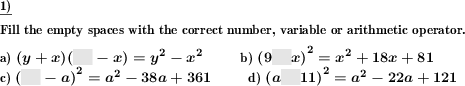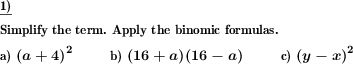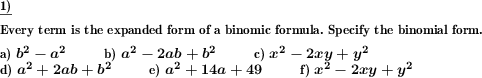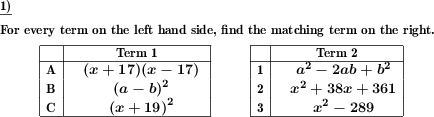Custom math worksheets at your fingertips# Details for problem "Binomic formula fill in blanks"

Quickname: 5815

Elementary School, Primary School, Junior High School, Middle School, High School.

## Summary

Fill gaps correctly in binomic formulas

## Example## Description

A binomic formula is shown together with the expanded and simplified term. The binomic formula term contains a blank space where a number, variable or arithmetic operator has been concealed.

The gap has to be filled in with the correct number, variable or arithmetic operator.

The summands of the formula's factors will be either a variable and a literal number or both of them will be variables. In no case, both summands will be numbers, which means the expanded term will always contain variables and it does not simplify to a single literal number.

The number of problems is selectable. It can be chosen what categories of

• numbers
• variables
• arithmetic operators

Variables that appear will always be named a and b or x and y.

Download free printable worksheets for this math problem here. The worksheet contains the problems only, the solution sheet includes the answers. Just click on the respective link.

•Worksheet 1Solution sheet with answers
•Worksheet 2Solution sheet with answers
•Worksheet 3Solution sheet with answers

If you can not see the solution sheets for download, they may be filtered out by an ad blocker that you may have installed. If this is the case, please allow ads for this page and reload the page. The solution sheets will then reappear.

• Do these sample worksheets do not really fit?
• Do you need more math worksheets, with a different level of difficulty?
• Would you like to combine different problems on a worksheet and adjust them to your needs?
• As a teacher, you can put together your own worksheets using the automatically generated math problems provided.
With a free initial credit, you can start creating your own math worksheets in a few minutes.

You can try it for free! Register here, to create custom worksheets now!

## Customization options for this problem

Parameter
Possible values
Number of problems
1, 2, 3, 4, 5, 6, 7, 8, 9, 10
Binomic formula types
(a+b)^2, (a-b)^2, (a+b)(a-b), (a+b)^2 & (a-b)^2, all
Fill in...
vars,numbers,operators, variables and numbers, arithmetic operators

## Similar problems

Remark
Description
In the form of a simplification task
Apply binomic formula expansion rules to simplify term.

## dw-Math worksheet templates that do contain this problem

Name
Title
Description
Binomic formulas: Expand and simplify
This worksheet provides basic exercises to become familiar with the binomic formulas.

## Other types of problems that appear on worksheets with this problem:

Relevance
Name
Description
Quickname
Example
****
Expand and simplify binomic formula
Apply binomic formula expansion rules to simplify term.****
Specify binomic formula for expanded term
A term is given which is the expanded form of a binomic formula. The original binomic formula has to be found.****
Match binomic formula to simplified form
Find matching terms of original and simplified binomic formulas in two lists.